# Maharashtra Board 12th Commerce Maths Solutions Chapter 2 Matrices Ex 2.6

Balbharati Maharashtra State Board 12th Commerce Maths Solution Book Pdf Chapter 2 Matrices Ex 2.6 Questions and Answers.

## Maharashtra State Board 12th Commerce Maths Solutions Chapter 2 Matrices Ex 2.6

Question 1.
Solve the following equations by the method of inversion:
(i) x + 2y = 2, 2x + 3y = 3
Solution:
The given equations can be written in the matrix form as: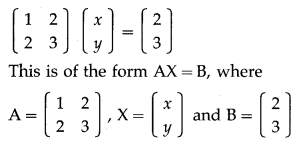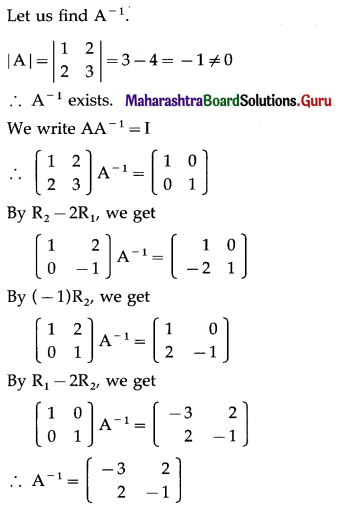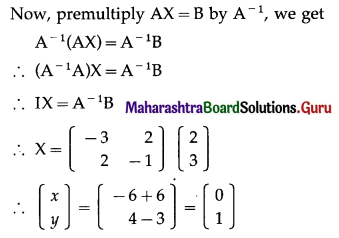By equality of matrices,
x = 0, y = 1 is the required solution.

(ii) 2x + y = 5, 3x + 5y = -3
Solution: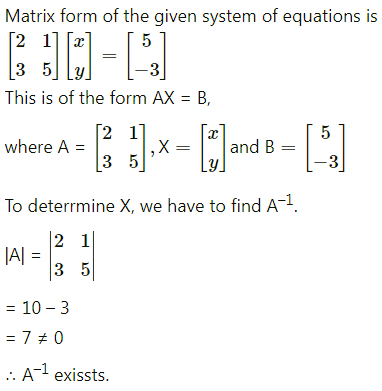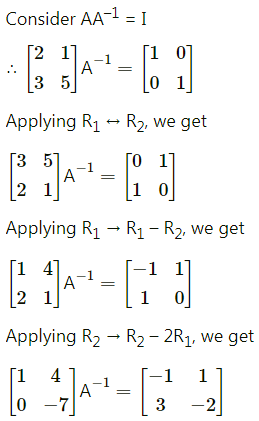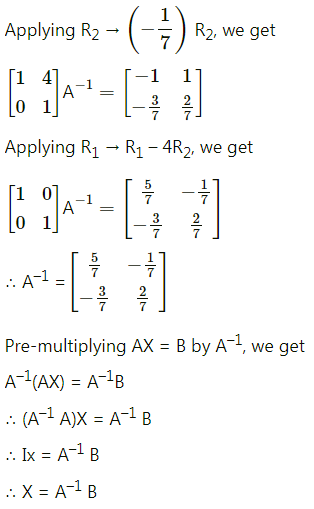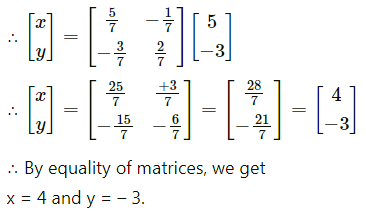(iii) 2x – y + z = 1, x + 2y + 3z = 8 and 3x + y – 4z = 1
Solution:
The given equations can be written in the matrix form as: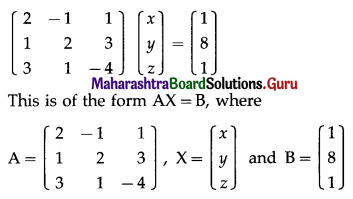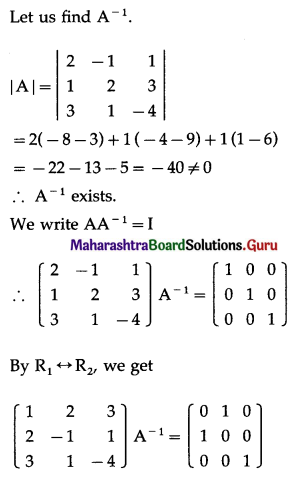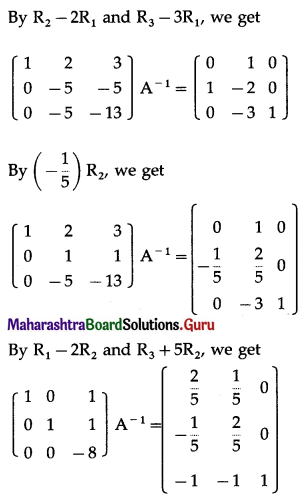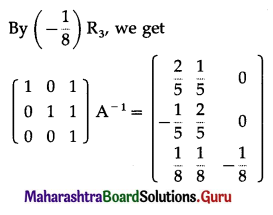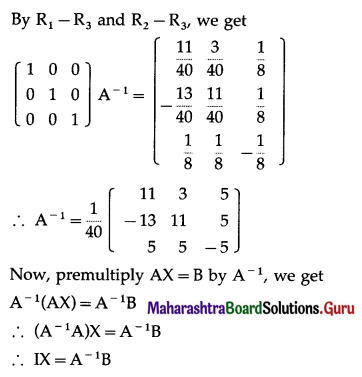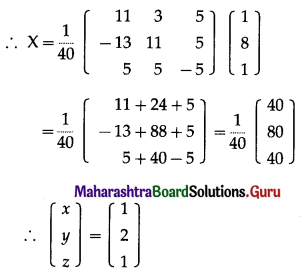By equality of matrices,
x = 1, y = 2, z = 1 is the required solution.

(iv) x + y + z = 1, x – y + z = 2 and x + y – z = 3
Solution: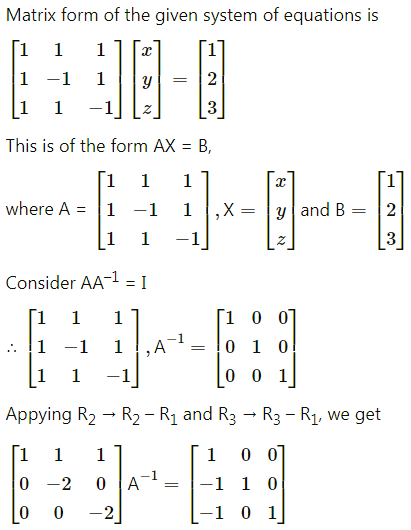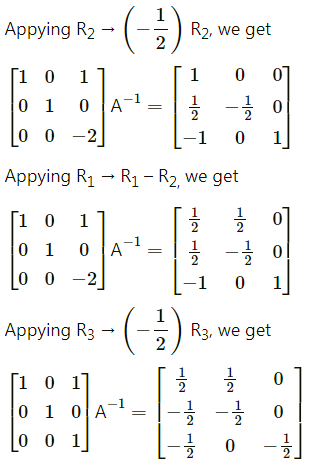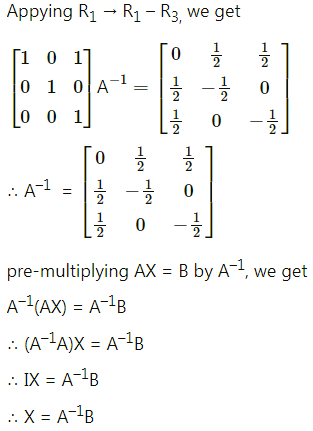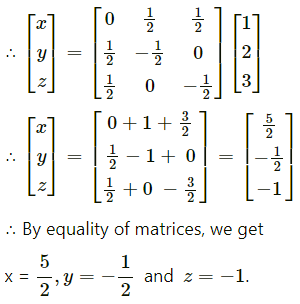Question 2.
Express the following equations in matrix form and solve them by method of reduction:
(i) x + 3y = 2, 3x + 5y = 4.
Solution:
The given equations can be written in the matrix form as: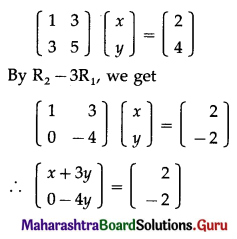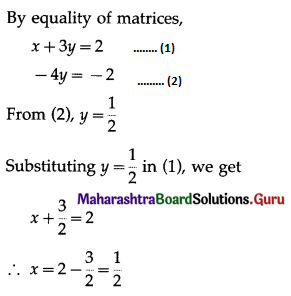Hence, x = $$\frac{1}{2}$$, y = $$\frac{1}{2}$$ is the required solution.

(ii) 3x – y = 1, 4x + y = 6.
Solution:
The given equations can be written in the matrix form as: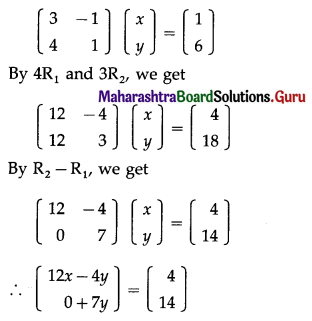By equality of matrices,
12x – 4y = 4 …..(1)
7y = 14 …..(2)
From (2), y = 2
Substituting y = 2 in (1), we get
12x – 8 = 4
∴ 12x = 12
∴ x = 1
Hence, x = 1, y = 2 is the required solution.(iii) x + 2y + z = 8, 2x + 3y – z = 11 and 3x – y – 2z = 5.
Solution:
The given equations can be written in the matrix form as: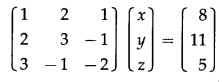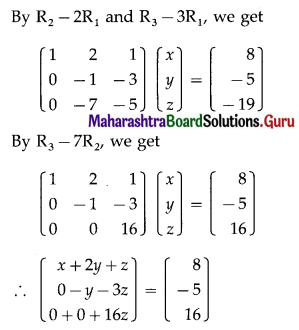By equality of matrices,
x + 2y + z = 8 ……(1)
-y – 3z = -5 …….(2)
16z = 16 ……….(3)
From (3), z = 1
Substituting z = 1 in (2), we get
-y – 3= -5
∴ y = 2
Substituting y = 2, z = 1 in (1), we get
x + 4 + 1 = 8
∴ x = 3
Hence, x = 3, y = 2, z = 1 is the required solution.

(iv) x + y + z = 1, 2x + 3y + 2z = 2 and x + y + 2z = 4.
Solution:
The given equations can be written in the matrix form as: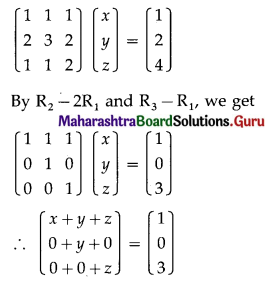By equality of matrices,
x + y + z = 1 ……(1)
y = 0
z = 3
Substituting y = 0, z = 3 in (1), we get
x + 0 + 3 = 1
∴ x = -2
Hence, x = -2, y = 0, z = 3 is the required solution.Question 3.
The total cost of 3 T.V. and 2 V.C.R. is ₹ 35000. The shopkeeper wants a profit of ₹ 1000 per T.V. and ₹ 500 per V.C.R. He sells 2 T.V. and 1 V.C.R. and he gets total revenue of ₹ 21500. Find the cost and selling price of T.V. and V.C.R.
Solution:
Let the cost of each T.V. be ₹ x and each V.C.R. be ₹ y.
Then the total cost of 3 T.V. and 2 V.C.R. is ₹ (3x + 2y) which is given to be ₹ 35000.
∴ 3x + 2y = 35000
The shopkeeper wants a profit of ₹ 1000 per T.V. and ₹ 500 per V.C.R.
The selling price of each T.V. is ₹ (x + 1000) and of each V.C.R. is ₹ (y + 500).
∴ selling price of 2 T.V. and 1 V.C.R is
₹ [2(x + 1000) + (y + 500)] which is given to be ₹ 21500.
∴ 2(x + 1000) + (y + 500) = 21500
∴ 2x + 2000 + y + 500 = 21500
∴ 2x + y = 19000
Hence, the system of linear equations is
3x + 2y = 35000
2x + y = 19000
The equations can be written in matrix form as: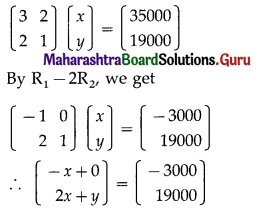By equality of matrices,
-x = -3000 …….(1)
2x + y = 19000 ……….(2)
From (1), x = 3000
Substituting x = 3000 in (2), we get
2(3000) + y = 19000
∴ y = 19000 – 6000 = 13000
Hence, the cost price of one T.V. is ₹ 3000 and of one V.C.R. is ₹ 13000 and the selling price of one T.V. is ₹ 4000 and of one V.C.R. is ₹ 13500.Question 4.
The sum of the cost of one Economics book, one Cooperation book, and one Account book is ₹ 420. The total cost of an Economic book, 2 Cooperation books, and an Account book is ₹ 480. Also, the total cost of an Economic book, 3 Cooperation books, and 2 Account books is ₹ 600. Find the cost of each book.
Solution:
Let the cost of 1 Economic book, 1 Cooperation book and 1 Account book be ₹ x, ₹ y and ₹ z respectively.
Then, from the given information
x + y + z = 420
x + 2y + z = 480
x + 3y + 2z = 600
These equations can be written in matrix form as: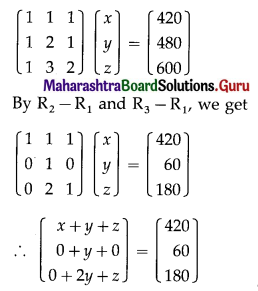By equality of matrices,
x + y + z = 420 …….(1)
y = 60
2y + z = 180 ………(2)
Substituting y = 60 in (2), we get
2(60) + z = 180
∴ z = 180 – 120 = 60
Substituting y = 60, z = 60 in (1), we get
x + 60 + 60 = 420
∴ x = 420 – 120 = 300
Hence, the cost of each Economic book is ₹ 300, each Cooperation book is ₹ 60 and each Account book is ₹ 60.